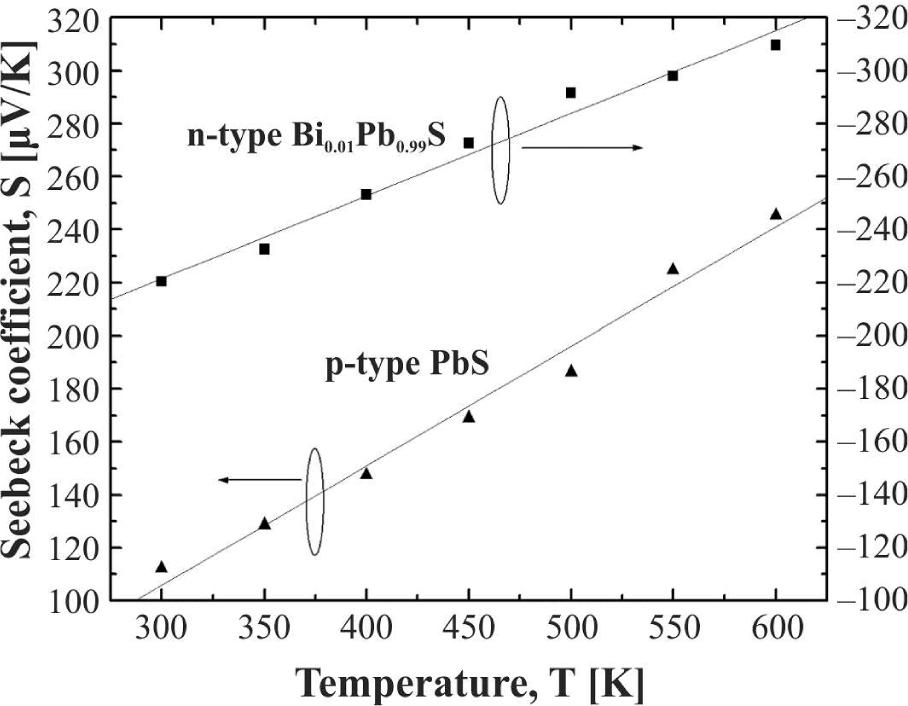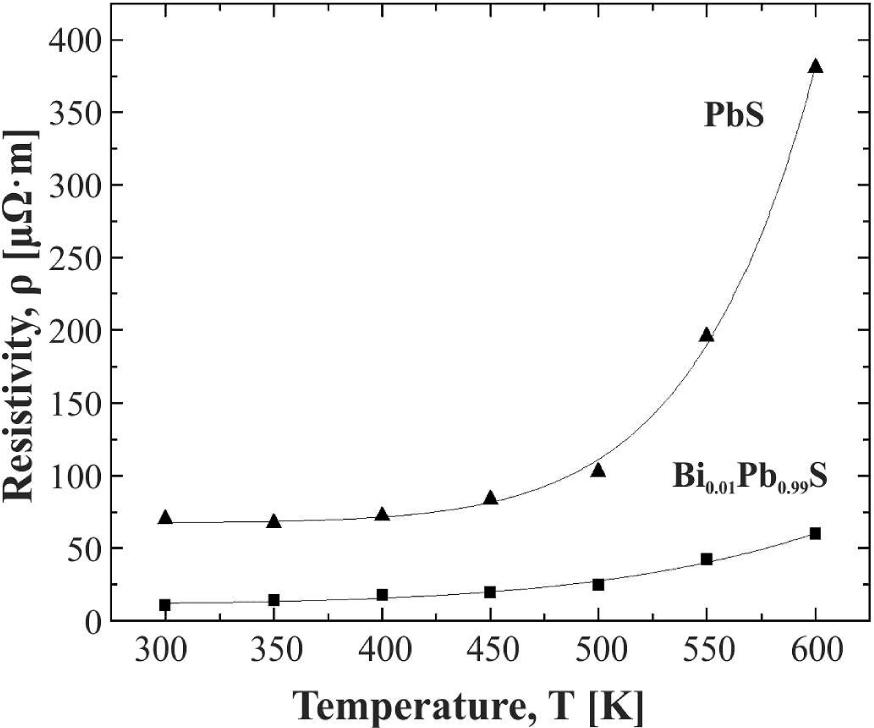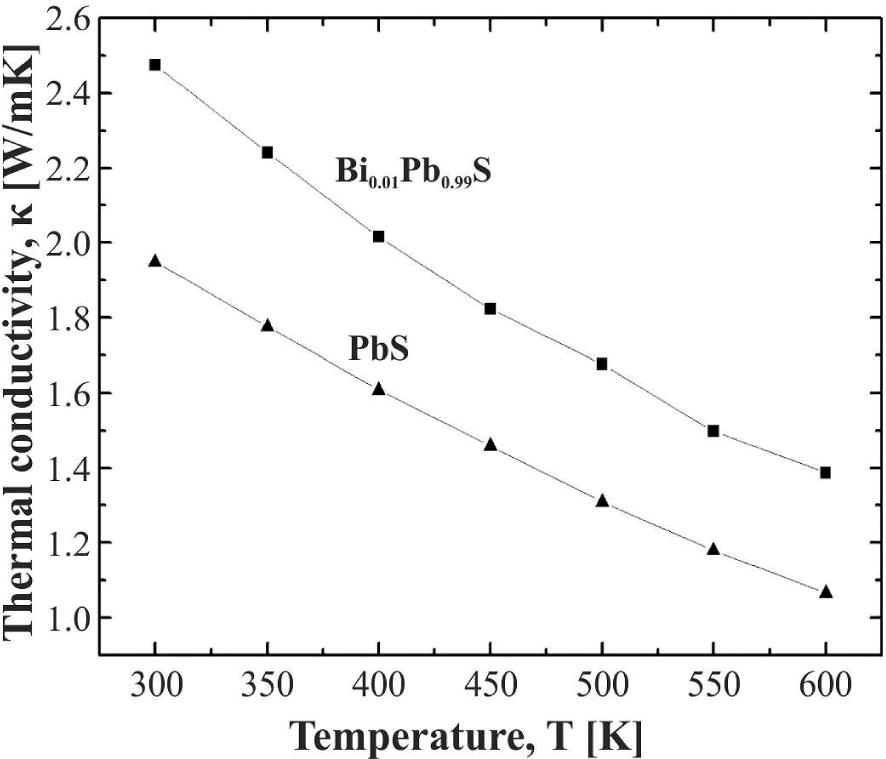Journal DetailsFormat
Journal
eISSN
2083-134X
First Published
16 Apr 2011
Publication timeframe
4 times per year
Languages
English
Open Access

# Hydrothermal synthesis and thermoelectric properties of PbS

###### Accepted: 28 Aug 2016
Journal DetailsFormat
Journal
eISSN
2083-134X
First Published
16 Apr 2011
Publication timeframe
4 times per year
Languages
English

In this paper, hydrothermal approach combined with high pressure sintering method was employed to synthesize PbS. The X-ray diffraction results show that single phase PbS can be obtained by a simple hydrothermal method. The scanning electron microscope results show that the PbS sample has nearly cubic shape and preserves well crystallized and coarse grains after high pressure sintering. The thermoelectric performance of PbS obtained in this study is comparable to that of a PbS sample prepared by conventional method. The carrier type and concentration of PbS can be tuned effectively by doping with Bi. The maximum figure of merit for PbS doped with 1 mol% Bi reaches 0.44 at 550 K, which is about 30 % higher than that of undoped PbS. These results indicate that hydrothermal method provides a viable and controllable way of tuning the electrical transport and thermoelectric properties for PbS.

#### Keywords

Introduction

Thermoelectric materials capable of converting energy between heat and electricity are currently attracting significant attention as a part of a search for sustainable alternative energy sources [1-6]. The efficiency of thermoelectric devices is strongly associated with the dimensionless figure of merit ZT defined as:

$ZT=(S2σ/κ)T$$$ZT = ({S^2}\sigma /\kappa )T$$

where T is temperature [K], S is Seebeck coefficient, σ is electrical conductivity, and κ is total thermal conductivity. Therefore, a combination of a high power factor (S2σ) and low thermal conductivity is an effective way to achieve high-performance thermoelectric materials.

The rocksalt-structured lead-based chalcogenides, have been extensively studied as promising thermoelectric materials [1, 6-9]. Especially lead telluride (PbTe), as one of the most frequently investigated materials in this system, remains the top-performing thermoelectric at midtemperature range (600 K to 900 K) . However, Te is a scarce element in the crust of the earth (0.001 ppm by weight). Lead sulfide (PbS) is much less expensive than its analogue PbTe. It is an ideal candidate for widespread application of environmentally stable and affordable thermoelectric material system provided its thermoelectric performance is comparable to that of PbTe. In order to enhance the thermoelectric properties of PbS, a lot of methods have been used, such as heavy doping , valence-band offset engineering and introducing nanocrystalline inclusions .

Metal sulfides are particularly difficult to synthesize mainly because of volatility of sulfur and its tendency to oxidize. This requires synthesis to be performed in a sealed tube under vacuum conditions. Usually, a conventional method of vacuum melting requires a long-duration heating process. Compared to it, the hydrothermal method is low cost and convenient for operating, what has been used for synthesis of many thermoelectric materials such as PbTe [12, 13], Bi2Te3 [14-16] and CoSb3 . As far as we know, there is little reported on the thermoelectric performance of hydrothermal synthesis of PbS [18, 19].

In this paper, both PbS and Bi doped PbS were synthesized by a simple hydrothermal synthesis method and sintered by high pressure method. The temperature dependence of thermoelectric properties of these compounds was studied.

Experimental

All the chemicals were analytically pure. In a typical synthesis procedure for PbS and Bi doped PbS (BixPb1−XS), NaOH (9.6 g) and NaBH4 (2 g) were dissolved in distilled water (80 mL) in a 200 mL Teflon-lined autoclave. Pb(NO3)2 (20 × (1 – x) mmol) were dissolved in distilled water (80 mL) in a 100 mL beaker. An ultrasonic cleaning machine was employed to facilitate the dissolution of starting materials introduced before. Sulfur powder (20 mmol) was put into the autoclave; after that, BiCl3 (20 × x mmol) was added. Then the solution from the beaker was transferred to the autoclave, and the reaction took place. Finally, the autoclave was sealed and placed in an oven at 453 K for 12 hours, then cooled naturally to room temperature. The product was filtered and washed with distilled water and ethanol several times, and dried in vacuum at 333 K overnight. The collected PbS and Bi doped PbS powder were shaped and sintered in a cubic anvil high-pressure apparatus (SPD 6 × 1200) at 1000 K and 2 GPa. The temperature and pressure were maintained for 20 min. After that, the electrical power for the heating was cut off and the pressure was released.

The phase structure of the products was characterized by X-ray diffraction (XRD) using a D8 advanced diffractometer with CuKα radiation (λ = 0.15406 nm). The morphology and microstructure studies and energy dispersive X-ray (EDX) analysis of the products were carried out by using a JXA-8200 scanning electron microscope (SEM). Hall coefficient was measured using the van der Pauw method with a constant magnetic field of 0.53 T and the electrical current of 20 mA. The carrier concentration was calculated from the Hall coefficient, assuming a single carrier model as a Hall scattering factor of unity. The Seebeck coefficient and electrical resistivity were measured from 300 K to 600 K by LSR-3. The thermal conductivity (κ) was calculated by using:

$κ=DρCp$$$\kappa= D\rho {C_p}$$

where D is the thermal diffusivity measured by the laser flash method (Netzsch LFA457), ρ is the density which was measured by Archimedes principle. Cp is specific heat capacity which was estimated from Dulong-Petit limit.

Results and discussion

Fig. 1 shows the XRD pattern of hydrothermally synthesized PbS powder. All the diffraction peaks can be perfectly indexed to NaCl structure without any noticeable second phase within the detection limit of the XRD equipment. The calculated lattice constant is about 5.913 Å which matches very well with the result of 5.914 Å in the reference (JCPDS Card File: PbS No. 78-1054). The SEM image in Fig. 2 shows that the crystals have a nearly cubic shape which further confirms the presence of cubically structured PbS. The energy dispersive spectrometer (EDS) measurement result shows that the atom fraction of Pb/S is about 1:1.02 for a hydrothermally synthesized sample. These results indicate that pure phase PbS was obtained via the simple hydrothermal synthesis method.

To identify the thermoelectric properties of PbS, high pressure method was employed to sinter the bulk sample. The XRD pattern of high pressure sintered bulk sample is shown in Fig. 3. It can be seen that it is still a single phase PbS. The SEM image of the sample (Fig. 4) shows that it still contains well crystallized and coarse grains, and the void-free structure is consistent with its relatively high density (∼97.7 %). Transgranular fractures and rectangular fractural surfaces are characteristic of the cubic structure of PbS. The measurement results of the electrical transport properties of PbS are shown in Table 1. It can be seen that the carrier concentration of PbS is about 7.36 × 1018 cm−3. The value is slightly higher than 1.2 × 1018 cm−3 of the sample prepared by conventional vacuum melting and quenching reported by Pei et al. . It can be attributed to different atom fractions in the samples prepared by different methods. But according to Mahan et al. , the carrier concentration is still much lower than in good thermoelectric materials.

Electrical transport properties of PbS and Bi doped PbS samples (BixPb1−xS) at room temperature.

SampleCarrier concentration [1018 cm−3]Hall mobility [cm2/V·s]Hall coefficient RH [cm−3]Resistivity [10−3 Ω·cm]Seebeck coefficient S [μV/K]
PbS7.3694.120.859.02220.44
Bi0.005Pb0.995S26.313.313−0.2471.61−134.29
Bi0.01Pb0.99S45.215133.99−0.141.03−112.27
Bi0.0l5Pb0.985S45.387126.83−0.141.08−48.48

In order to tune the carrier concentration of PbS, Bi was doped into PbS as a donor.

The XRD patterns of PbS doped with Bi (BixPb1−xS, x = 0, 0.005, 0.01, 0.015) are shown in Fig. 3. It can be seen that all the samples are single phase when the content of additive Bi is lower than 1.5 mol% (x < 0.015). The impurity phase is found in BixPb1−xS, when x = 0.015. These results indicate that the solubility of Bi in PbS is lower than 1.5 %.

Table 1 shows the electrical transport properties of Bi doped PbS samples (BixPb1−xS) at room temperature. The value of Hall coefficient changes from positive to negative, which indicates that the conductivity type of PbS changes from p to n after doping with Bi. The carrier concentration of PbS increases with an increase of Bi content but the carrier concentration value of Bi0.015Pb0.985S is similar to that of Bi0.01Pb0.99S. These results indicate that the incorporative rate of Bi in PbS is about 1.0 mol%, which is consistent with the XRD results in Fig. 3. The Bi0.01Pb0.99S sample has the lowest resistivity due to its high carrier concentration and large Hall mobility. The Seebeck coeffi-cient changes to negative which is consistent with the Hall coefficient value. The absolute value of Seebeck coefficient decreases with the increase in Bi content. The largest power factor was also obtained for Bi0.01Pb0.99S sample.

The temperature dependences of thermoelectric properties including Seebeck coefficient, resistivity and thermal conductivity of PbS and Bi0.01Pb0.99S were measured from 300 K to 600 K. As seen from Fig. 5, the absolute values of Seebeck coefficient for PbS and Bi0.01Pb0.99S show approximately a linear relationship in the measured temperature range. The slope for Bi0.01Pb0.99S is lower than that for PbS. The diffusive part of the Seebeck coefficient (T-linear part) can be described by the formula :Temperature dependence of Seebeck coefficient for PbS and Bi0.01Pb0.99S.$S∝π2κB2T/eEF$$$s \propto {\pi ^2}\kappa _B^2T/e{E_F}$$

where κB, EF, e are the Boltzmann constant, Fermi level and electron charge, respectively. This formula indicates that the slope of the plot S vs. T is inversely proportional to the Fermi level. Hence, the reduction of the slope of the plot S vs. T for Bi doped PbS would reflect the rise of EF, which is consistent with the increased carrier concentration for Bi doped PbS.

Fig. 6 shows the temperature dependence of electrical resistivity for PbS and Bi0.01Pb0.99S. The electrical resistivity for both samples increases with the increase of temperature, which is the typical behavior of degenerate semiconductors. The temperature dependence of resistivity can be described well by the power law:Temperature dependence of electrical resistivity for PbS and Bi0.01Pb0.99S.$ρ=ρ0+aTγ$$$\rho= {\rho _0} + a{T^\gamma }$$

The values of ρ0 and γ obtained from the above formula, are shown in Table 2. The power law dependence of the electrical resistivity results from the phonon scattering of charge carriers .

The values of ρ, a and γ obtained from the formula ρ = ρ0+aTγ.

Sampleρ [10 −6Ω·cm]a(10−16)γ
PbS67.552.6810.82
Bi0.01Pb0.99S11.434.826.12

Fig. 7 presents the power factors of PbS and Bi0.01Pb0.99S derived from the electrical conductivities and Seebeck coefficients as a function of temperature. it can be seen that the power factor is higher as Bi is doped in PbS. The power factors of PbS and Bi0.01Pb0.99S show maximum values of 8.88 and 14.57 μW·cm−1·K−2, respectively. Compared to PbS, the maximum power factor peaks of Bi0.01Pb0.99S increased by 64.07 %.Temperature dependence of power factor for PbS and Bi0.01Pb0.99S.

Fig. 8 shows the temperature dependence of thermal conductivities for PbS and Bi0.01Pb0.99S. Generally, the total thermal conductivity has two components, the lattice part κph and the electronic part κe. κe can be simply estimated through the Wiedemann-Franz law:Temperature dependence of thermal conductivity for PbS and Bi0.01Pb0.99S.$κe=LσT$$${\kappa _e} = L\sigma T$$

where L is the Lorenz number. The Lorenz number is estimated by the equation:

$L=1.5+exp⁡[−S/116]$$$L = 1.5 + \exp [ - \left| S \right|/116]$$

which was proposed by Snyder et al. . The total thermal conductivity of Bi0.01Pb0.99S is higher than that of PbS in the measured temperature range due to its high κe. The κph is nearly the same for both samples considering the measurement errors. The κph values of both the samples are about 2.0 W·m−1·K−1, which is much smaller than that of PbS prepared by Pei et al.  (∼2.5 W·m−1·K−1) and Wang et al.  by other conventional methods. This difference can be attributed to the grain boundaries and crystal defects resulting from hydrothermal process and high pressure compression.

Using the measured electrical transport properties and thermal conductivity, the figure of merit, ZT was calculated. The temperature dependence of ZT is presented in Fig. 9. The maximum ZT value for the PbS is about 0.32 at 500 K, which is about 40 % higher than that of the sample prepared by vacuum melting reported by Pei et al.  at the same temperature. In addition, the hydrothermal synthesis and high pressure sintering used in this work is much faster and the technique is very easy compared to the conventional methods. The ZT values of Bi0.01Pb0.99S are higher than that of PbS in the measured temperature range. The maximum ZT for Bi0.01Pb0.99S is about 0.44 at 550 K, which is about 30 % higher than that of undoped PbS. Further enhanced ZT value of PbS based systems can be expected by depressing its thermal conductivity via introducing nanoinclusions.

Conclusions

Single phase PbS was prepared successfully by a simple hydrothermal method. High density bulk samples were obtained by high pressure sintering. The electrical transport and thermoelectric properties of PbS obtained by hydrothermal and high pressure sintering are comparable to the properties of PbS compounds prepared by conventional methods. The low level substitution of Bi (⩽1.5 mol%) for Pb in PbS introduces effective electron donors in the lattice and considerably increases the carrier concentration. The maximum figure of merit for PbS doped with 1 mol% Bi reaches 0.44 at 550 K, which is about 30 % higher than that of undoped PbS.

#### The values of ρ, a and γ obtained from the formula ρ = ρ0+aTγ.

Sampleρ [10 −6Ω·cm]a(10−16)γ
PbS67.552.6810.82
Bi0.01Pb0.99S11.434.826.12

#### Electrical transport properties of PbS and Bi doped PbS samples (BixPb1−xS) at room temperature.

SampleCarrier concentration [1018 cm−3]Hall mobility [cm2/V·s]Hall coefficient RH [cm−3]Resistivity [10−3 Ω·cm]Seebeck coefficient S [μV/K]
PbS7.3694.120.859.02220.44
Bi0.005Pb0.995S26.313.313−0.2471.61−134.29
Bi0.01Pb0.99S45.215133.99−0.141.03−112.27
Bi0.0l5Pb0.985S45.387126.83−0.141.08−48.48

Girard S.N., Schmidt Rohr K., Chasapis T.C., Adv. Funct. Mater., 23 (2013), 747.GirardS.N.Schmidt RohrK.ChasapisT.C.Adv. Funct. Mater23201374710.1002/adfm.201201944Search in Google Scholar

Snyder G.J., Toberer E.S., Nat. Mater., 7 (2008), 105.SnyderG.J.TobererE.S.Nat. Mater7200810510.1038/nmat209018219332Search in Google Scholar

Heremans J.P., Jovovic V., Toberer E.S., Science, 321 (2008), 554.HeremansJ.P.JovovicV.TobererE.S.Science321200855410.1126/science.115972518653890Search in Google Scholar

Urban J.J., Talapin D.V., Shevchenko E.V., Nat. Mater., 6 (2007), 115.UrbanJ.J.TalapinD.V.ShevchenkoE.V.Nat. Mater6200711510.1038/nmat182617237786Search in Google Scholar

Disalvo F.J., Science, 285 (1999), 703.DisalvoF.J.Science285199970310.1126/science.285.5428.70310426986Search in Google Scholar

Lee Y., Lo S.H., Chen C., Nat. Commun., 5 (2014), 4640.LeeY.LoS.H.ChenC.Nat. Commun52014464010.1038/ncomms690225533202Search in Google Scholar

Ravich I.I., Semiconducting Lead Chalcogenides, Springer US, New York, 2013.RavichI.I.Semiconducting Lead ChalcogenidesSpringer USNew York2013Search in Google Scholar

Zhao L.D., He J., Hao S., J. Am. Chem. Soc., 134 (2012), 16327.ZhaoL.D.HeJ.HaoS.J. Am. Chem. Soc13420121632710.1021/ja306527n22991921Search in Google Scholar

Wu H., Carrete J., Zhang Z., NPG Asia. Mater., 6 (2014), e108.WuH.CarreteJ.ZhangZ.NPG Asia. Mater62014e10810.1038/am.2014.39Search in Google Scholar

Liu W., Lukas K C., Mcenaney K., Energ. Environ. Sci., 6 (2013), 552.LiuW.LukasK C.McenaneyK.Energ. Environ. Sci6201355210.1039/C2EE23549HSearch in Google Scholar

Wang H., Schechtel E., Pei Y., Adv. Energ. Mater.,3 (2013), 488.WangH.SchechtelE.PeiY.Adv. Energ. Mater3201348810.1002/aenm.201200683Search in Google Scholar

Kim H.S., Gibbs Z.M., Tang Y., Snyder G.J., APL Mater., 3 (2015), 041506.KimH.S.GibbsZ.M.TangY.SnyderG.J.APL Mater3201504150610.1063/1.4908244Search in Google Scholar

Zhu T.J., Chen X., Cao Y Q., J. Phys. Chem. C, 113 (2009), 8085.ZhuT.J.ChenX.CaoY Q.J. Phys. Chem. C1132009808510.1021/jp900452bSearch in Google Scholar

Zhao X., Ji X., Zhang Y., Appl. Phys. Lett., 86 (2005), 062111.ZhaoX.JiX.ZhangY.Appl. Phys. Lett86200506211110.1063/1.1863440Search in Google Scholar

Cao Y., Zhao X., Zhu T., Appl. Phys. Lett., 92 (2008), 3106.CaoY.ZhaoX.ZhuT.Appl. Phys. Lett922008310610.1063/1.2898711Search in Google Scholar

Tang X., Xie W, Li H., Appl. Phys. Lett., 90 (2007), 12102.TangX.XieWLiH.Appl. Phys. Lett9020071210210.1063/1.2425007Search in Google Scholar

Alboni P., Ji X., He J., J. Appl. Phys., 103 (2008), 113707.AlboniP.JiX.HeJ.J. Appl. Phys103200811370710.1063/1.2937904Search in Google Scholar

Johnsen S., He J., Androulakis J., J. Am. Chem. Soc., 133 (2011), 3460.JohnsenS.HeJ.AndroulakisJ.J. Am. Chem. Soc1332011346010.1021/ja109138pSearch in Google Scholar

Kuang D., Xu A., Fang Y., Adv. Mater., 15 (2003), 1747.KuangD.XuA.FangY.Adv. Mater152003174710.1002/adma.200304623Search in Google Scholar

Pei Y.L., Liu Y., J. Alloy. Compd., 514 (2012), 40.PeiY.L.LiuY.J. Alloy. Compd51420124010.1016/j.jallcom.2011.10.036Search in Google Scholar

Mahan G., Solid State Phys., 51 (1997), 81.MahanG.Solid State Phys5119978110.1016/S0081-1947(08)60190-3Search in Google Scholar

Li D., Yang K., Hng H., J. Appl. Phys., 104(2008), 103720.LiD.YangK.HngH.J. Appl. Phys104200810372010.1063/1.3029694Search in Google Scholar

• #### Growth, spectral, optical, dielectric and third order nonlinear studies on 2-amino 4-methylpyridinium salicylate single crystals

Recommended articles from Trend MD